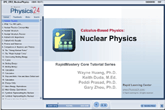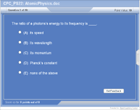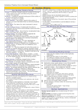How to Learn in 24 Hours?

 Need Help? M-F: 9am-5pm(PST): Toll-Free: (877) RAPID-10 or 1-877-727-4310 24/7 Online Technical Support: The Rapid Support Center vip@rapidlearningcenter.com Secure Online Order:Need Proof? Testimonials by Our Users

 Rapid Learning Courses: MCAT in 24 Hours (2021-22) USMLE in 24 Hours (Boards) Chemistry in 24 Hours Biology in 24 Hours Physics in 24 Hours Mathematics in 24 Hours Psychology in 24 Hours SAT in 24 Hours ACT in 24 Hours AP in 24 Hours CLEP in 24 Hours DAT in 24 Hours (Dental) OAT in 24 Hours (Optometry) PCAT in 24 Hours (Pharmacy) Nursing Entrance Exams Certification in 24 Hours eBook - Survival Kits Audiobooks (MP3)

 Tell-A-Friend: Have friends taking science and math courses too? Tell them about our rapid learning system.Home »  Physics »  Calculus Physics

Nuclear Physics

 Topic Review on "Title": Atomic Number:  The number equal to the number of protons in an atom that determines its chemical properties. Symbol: Z Atomic Mass: The mass of an atom expressed in atomic mass units. Strong (nuclear) Force: A fundamental force that is associated with the strong bonds between quarks and other subatomic particles. Weak (nuclear) Force:  One of the four fundamental forces that is associated with nuclear decay.  Binding Energy: The energy needed to separate the constituent parts of an atom or nucleus. Mass Defect: The difference between the mass of an atom and the sum of the masses of its individual components. Mass-Energy Equivalence:  All mass represents an equivalent amount of energy. 1 amu = 931 MeV. Radioactivity: Emission of radiation as a consequence of a nuclear reaction, or directly from the breakdown of an unstable nucleus.  Half Life:  The time required for half of the nuclei in a sample of a specific isotope to undergo radioactive decay. Alpha Particle: A positively charged helium nucleus (consisting of two protons and two neutrons). Beta Particle: An energetic electron produced as the result of a nuclear reaction or nuclear decay. Gamma Particle/Ray: Very high frequency electromagnetic radiation emitted as a consequence of radioactivity.  Fission: The process whereby one item splits to become two.

Rapid Study Kit for "Title":
 Flash Movie Flash Game Flash Card Core Concept Tutorial Problem Solving Drill Review Cheat Sheet"Title" Tutorial Summary : This tutorial specifically describe the structure and behavior of the atomic nucleus.  The nucleus is composed of neutrons and protons.  Obviously the similarly charged protons repel each other, but nuclear forces usually overcome that tendency and the nucleus is kept intact.  However, sometimes that repulsion is too great and the nucleus crumbles apart.  This is the process of radioactivity.  Alpha, beta, and gamma are the three types of nuclear radioactivity.  Each has different properties.  A nucleus may also break apart into roughly two equal pieces, this is the process of nuclear fission.

 Tutorial Features: Specific Tutorial Features: Animations depicting nuclear processes like fission and radioactivity. Step by step analysis of an example of the concepts of binding energy and mass defect. Series Features0: Concept map showing inter-connections of new concepts in this tutorial and those previously introduced. Definition slides introduce terms as they are needed. Visual representation of concepts Animated examples—worked out step by step A concise summary is given at the conclusion of the tutorial.

 "Title" Topic List: Nuclear composition Notation Forces Binding energy Mass defect Isotopes Radioactive decay Alpha decay Beta decay Gamma decay Nuclear equations Examples of nuclear equations Half life Decay constant Decay formula and examples Applications of nuclear science

See all 24 lessons in high school  physics, including concept tutorials, problem drills and cheat sheets:
Teach Yourself Calculus Physics Visually in 24 Hours